Here's what we have so far.

Find the force(s) on an object.

Use Newton's 2nd Law to obtain the object's acceleration.

Use the kinematic equations to track the object's motion over time.

gravitational force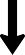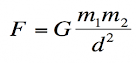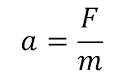Δx = vavgt               Δx = vit + ½ at2

vf = at + vi               vf2 = vi2 + 2aΔxElectromagnetic force

F = ?

We also need vi, at the least, to be able to do anything with these.

You're probably thinking to yourself, the whole gravitational force calculation seems pretty unnecessary, given that it's just going to lead to an acceleration of 9.8 m/s2.

And you're right, as long as you're focused on something falling freely near the Earth's surface. So if that's your focus, jump straight to a = 9.8 m/s2. And if you're focused on higher altitudes (including outer space), or objects on the Moon or Mars, then do the force calculation. BUT ... force calculations do become necessary when multiple forces are acting at once. In such cases (which comprise almost all cases), we must know all of the forces to be able to add them and find the net force; the acceleration would then be calculated from the net force.

Also, realize that finding the force as a step toward finding the acceleration is unnecessary if you have position vs time data for the object.

Providing you with this data, and having you plot it and "extract" the acceleration (and other quantities), was how we operated at first.

Let's put it (our "dynamics general strategy") all together in the analysis of a real scenario.

In 1971, Apollo 14 commander Alan Shepard used a make-shift golf club to hit a golf ball (mass = 0.046 kg) on the Moon's surface. Assuming the ball was given an initial velocity of 40 m/s at an angle of 20° with the ground, how far did the ball go?

Once the ball is launched, only a single force acts on it. The force of gravity. (This is the definition of a projectile.) Let's find that force.

Because this gravitational force is the only force acting on the ball, after it's launched, it is also the net force.

Thus, we can say Fnet = -0.07477 j (N). Yes, I'm attaching a -j to indicate direction.

Using the model a = F / m, we determine that a = (-0.07477 j) / 0.046 = -1.625 j (m/s2).

We wish to find the range of the ball, ∆x. I'm going to choose ∆x = vit + ½at2 as my model.

Considering that the ball's motion is best analyzed by tracking its horizontal and vertical motions separately (and we can do this because they are independent of one another), we must prepare to do so by resolving the initial velocity vector into its components.

vx = 40 cos(20°) = 37.588 i (m/s)

vy = 40 sin(20°) = 13.681 j (m/s)

You could write vi = (37.588 i) + (13.681 j), if you wanted to.

Now we can use our model, twice. Notice I am adopting x and y notation, to prevent confusion.

(Remember that acceleration is zero along the x-axis.)

∆x = (37.588 i)t

∆y = (13.681 j)t + ½(-1.625 j)t2

Do you remember how we determined range, using these two models?

First, we find the time associated with ∆y = 0. Then we solve for ∆x at that time.

0 = 13.681t - 0.8125t2 leads to 0 = 13.681 - 0.8125t, which gives us t = 16.838 seconds.

Finally, ∆x = (37.588)16.838 = 632.91 m.

The ball traveled 632.91 meters in the direction it was hit. (The lack of an atmosphere on the Moon would prevent curvature in flight. The frictionless environment would also allow the ball to travel very far. Compare the range of this golf ball to Tiger Woods' longest drive (in 2008) of 389 meters.)

Okay, we're going to have to work out how to determine electromagnetic forces (at least in certain cases) or we'll be stuck, only able to model projectiles and objects for which we're given all of the forces (unless we're able to collect force or position data).

As a first step, we'll play a game: Spot the forces. We'll look at photographs and try to identify all of the forces acting on some particular object. We're looking for instances where something is pushing or pulling on our object. And here's an important realization: every force you find (apart from gravity) will be some version of an electromagnetic force. Because that's all there is, outside the tiny nucleus of an atom. There's only gravity and electromagnetic forces.

Let's begin. Spot the forces on the boat.

Gravity is shown as a blue vector, pointing toward the Earth's center.

The other "pushes or pulls" are shown as red vectors. What do we have?

Well, the truck is certainly pulling the boat forward. The ground is pushing upward, supporting the boat's weight. And there is some air resistance or friction opposing its motion, pushing backwards.

(Notice we are only concerned with forces acting on the boat and not within the boat. Mentally breaking the boat into pieces, each of which exerts a force on the surrounding pieces, is unnecessary and counterproductive.)

We'll play again. Spot the forces on the wagon.

As always, we have gravity pulling downward (shown in blue).

The other forces (in red) are: the ground pushing up on the wagon, supporting its weight. (It pushes on all four wheels. To simplify, we'll group these together and consider a single upward push from the ground.) We also have the boy pulling on the wagon, and we have some amount of resistance or friction (much more from the ground than the air).

Okay, let's do another. Spot the forces on the penguin.

Yes, there's gravity. There's always gravity.

We also have the ground pushing on the penguin's belly, supporting its weight. And we have perhaps a tiny bit of resistance or friction (but not much, since we're on slippery snow).

I want to point out something important. In most scenarios, the ground will be supporting our object. (Obvious exceptions are for projectiles and items hanging from above.) This "supporting" force should be recognized and depicted as acting perpendicular to the ground. I did that above. The ground does not push "up" on the penguin. It pushes "up and to the right". It does not need to (and many times will not) directly oppose gravity.

Furthermore, in most cases, the force of friction will point opposite the direction of motion. Here, the penguin moves downhill, so the friction force points uphill.

One more. Spot the forces on the girl.

This one is pretty easy.

There's gravity. And there's the ropes, which we'll together count as a single force. (Technically, the seat is pushing on her, but I want to consider the seat as part of the girl. If we do that, then the ropes are pulling on her.) Is there friction of some sort? Yes, but it's so minimal that it's not worth considering. The only time we will not ignore air resistance is when something is moving, say, faster than 30 mph. Only when we think that excluding it will cause a large error in our analysis.mass of Moon = 7.348 x 1022 kg

radius of Moon = 1.737 x 106 m

For reference:

G = 6.67408x10-11 m3/(kg·s2)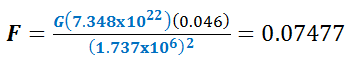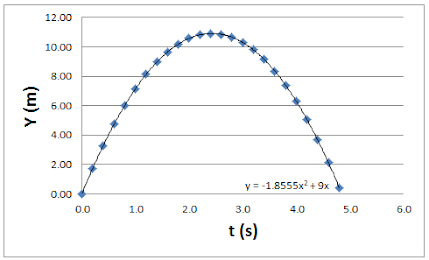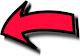An example of what we've done in the past.

Electromagnetic force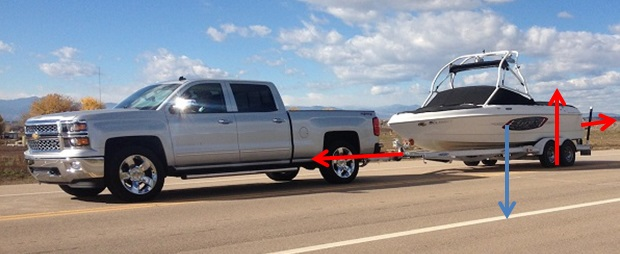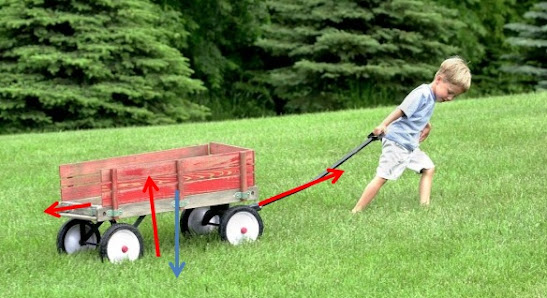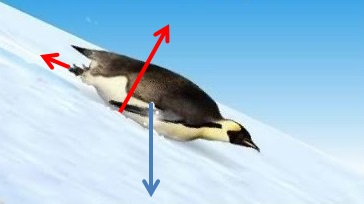The  many  faces  of  the

Electromagnetic  force

Of all the different ways that one object can push or pull on another, many instances occur often enough that it's very helpful to have a special name for them.

Thus, the word "friction", for example. Friction is what we call an electromagnetic force when the force opposes motion. Friction arises from one object sliding against another, or it can arise from an object moving through a fluid (a liquid or gas). When the fluid is air, we may call the friction by the more-descriptive name "air resistance". Down at the molecular level, it arises from the molecules of one substance pushing the molecules of another, by way of their electrons.

Do not think of friction as being some fundamental type of force in the universe. The word is just a useful way to refer to an electromagnetic force behaving in a specific way.

The "supporting" force referenced in three of the examples above also gets its own name, and I don't mean "supporting". When one object, even if it's the Earth, supports as least some of the weight of some object, we often refer to that force as either a "reaction force" or "normal force". These terms are not as well known as friction, at least among the general public.

Why the word "normal"? Because, in a mathematical context, "normal" means perpendicular. Remember this!

Actually, any time one object pushes into another, from the bottom or the side or from any angle, the force of contact can be called a normal force (as long as it acts perpendicular to the surfaces). Should a contact force not act perpendicular to the touching surfaces, it can be resolved into components, one of which DOES act perpendicular to the surfaces. This component could be called a normal force. (I won't ask you to do this.)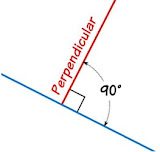Label normal force vectors as

FN or Fn or just N

Label frictional force vectors as

Ff

When a rope or something rope-like is exerting an electromagnetic force on an object, we typically call that electromagnetic force by the name "tension".

For the girl swinging on the tree swing, it is the forces of tension and gravity which act on her.

Tension forces always pull. They don't push.

Tension forces arise from the attractive electrical forces between molecules in a solid. Tension forces prevent a solid from being pulled apart, or at least they make it difficult.

Label tension force vectors as

Ft or just T

When a spring or something spring-like exerts an electromagnetic force on an object, we call that electromagnetic force by the name of "spring force".

Springs can both push and pull, depending on whether they are compressed or stretched from their equilibrium length.

The only reason why springs are singled out among all things that exert forces is because it is particularly easy to determine the force exerted by a spring. The simple formula (or model) goes by the name of Hooke's Law, and we'll examine it later.

Label spring force vectors as

Fs

What do you call the force from a hand, pushing on something? What do you call the force from a foot, kicking a ball?

It is very likely that these forces, in whole or part, are "normal". Yet many people prefer to call them by the name "applied force". The term is admittedly generic.

Most people treat any force arising from a person as "applied". Can a dog exert an applied force, though? Can a robot? Can a wall? It's not clear ... or at least consistent.

At this point, we have to consider whether the introduction of the term reduces confusion or increases it. Remember, "applied force" is not some fundamental type of force in the universe. It's just what people tend to call electromagnetic forces between a person's hand and some object, and in similar cases. (If you could accurately define "similar" in this case, you'd have cleared up the confusion!) It's a word made up to serve a function. Does it serve that function well? You can decide for yourself.

But, hear me! Never worry about whether a force is "applied" or not. If it's not clear, then call the force by what it is, such as "the force of my elbow pushing on the pillow", and label it as Felbow or whatever makes sense to you. If someone asks you, "Is this force applied?", feel free to say, "It's electromagnetic!"

Label applied force vectors as

Fa or Fapp

The electromagnetic force has many faces, but they are all, deep down, electromagnetic ... meaning what?

Meaning that they are the result of protons and electrons attracting and/or repelling one another due to their electric charge. It is simply a FACT of our universe that two objects with a negative electric charge (like electrons) will repel one another, and likewise a FACT that a negatively-charged object (again, an electron) will attract a positively-charged object (like a proton).

When my hand pushes on a door (an applied force!), it is actually the electrons in my hand pushing on the electrons in the door. But the electrons in the door also push on the ones in my hand. And so what happens is that, because I tried to force my electrons too close to another group of electrons, both sets repelled away from one another. But as the door swung away, I flexed my muscles and held my ground. I used my arm to exert a counteracting force on my hand, so that the net force on my hand was practically zero, and my hand did not accelerate away from the door. The door apparently lacked a corresponding force of sufficient strength.

Friction, tension, normal forces, springs ... it's all electrons (and sometimes protons).

Can electrons and protons interact by any other mechanism? Yes, gravity. And that's it, unless you want to include the nuclear forces.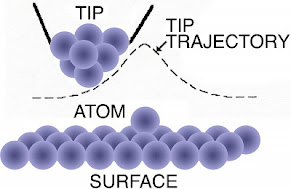A depiction of one group of atoms approaching another group of atoms. We can imagine the atoms as having an electron "cloud" surrounding them. The electrons around the "tip" will repel the electrons on the "surface".

Why don't the atoms in the surface (or tip) repel from one another? Ionic and/or covalent bonding.

(This picture is meant to illustrate the use of a scanning tunneling microscope, if you were curious.)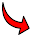When you need to model some part of the world, and you're interested in how it moves with time ...

begin with the forces.

• Identify them.
• Diagram them.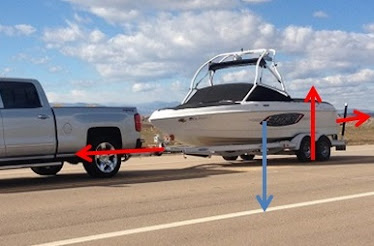and when it comes to

the gravitational force...

Label gravitational force vectors as

Fg or W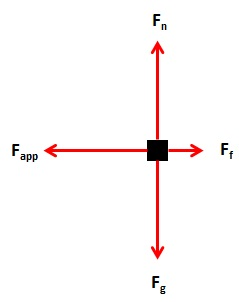Identify

diagram

Fg is the only one we know how to find, for now. Although, to find it, we need to know the boat's mass. Let's say that we do know it: it's 740 kg.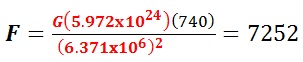9.8

Here's the next big step...

We can use Newton's Second Law to work out part of what remains!

Yes, this seems backwards. We're supposed to find the forces first, then use a = F / m.

Yet I am suggesting using a = F / m to find an unknown force. It's actually not that strange.

Here's how it works:

We must work in x and y, i and j.

Let's say that, by watching the boat, we can tell that it's speeding up. While we may not be able to tell the magnitude of its acceleration, we can at least tell its direction. The boat is NOT accelerating upwards, or downward, or backwards. It's accelerating forwards. Thus, we know that, using components:

ax = does not equal zero

ay = 0 j (m/s2)

Because ax depends solely on Fx, and ay depends solely on Fy, we can then say,

net Fx = m ax = does not equal zero

net Fy = 740(0 j) = 0 j (N)

Now, we're almost finished.

We know that the net Fy = 0 j (N). We also know that the net Fy = Fn + Fg. Right? There are only two vertical forces, and we add them to get the net vertical force.

So, given that Fg = -7252 j (N), and net Fy = 0 j (N), and net Fy = Fn + Fg,

then 0 j = Fn + (-7252 j), which can be solved to find that Fn = 7252 j (N).

Now, I've gone step-by-step here to teach the underlying concept. But if you just stop for a moment and think, if something is not accelerating vertically, then the "force up" has to equal the "force down".

IF ... and this is a big if ... there is only one force up (the normal) and one force down (gravity or weight, same thing), then the normal force will simply equal the weight (gravity), except with the opposite sign.

Great! So now we know both Fn and Fg. What about Fapp and Ff?

Well, it turns out that Ff is simply proportional to Fn. This relationship is known from careful observation and data analysis. So finding Ff will be easy. And calculating Fapp will be ... well, pretty much impossible. Sorry. So someone will always have to give it to you.* Or, out in the real world, you'll have to measure it. Unless you elect to jump straight to measuring the acceleration. I suppose it depends on the tools at your disposal and what you're actually trying to accomplish. Also... of course, there are times when there is no applied force. That's always nice.

*If the teacher gives Fapp to you, how did he or she find it? It was probably just made up, to teach you the technique of solving problems. Nothing wrong with that.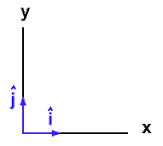I re-wrote a = F / m as F = ma.

"W" for weight

This type of diagram is traditionally called a "free body diagram" or FBD, although you might hear "force diagram". You should get used to these terms.

I'd recommend spending a few minutes skimming the Wikipedia page on free body diagrams.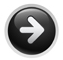I originally planned to continue developing this online tutorial, but my attention has shifted elsewhere. Looks like this is where it'll end...  -- Mr. Perrone

Forces

Kinematics